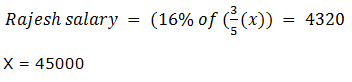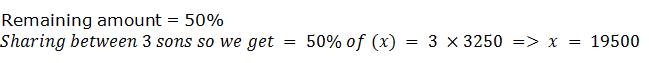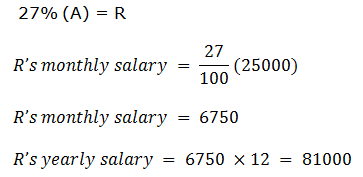## Percentage For SBI PO : Set – 16

1) Rajesh decided to donate 16% of his salary on the day of donation he changed his mind and donated Rs. 4320 which was three-fifths of what he had decided earlier. How much is the salary of Rajesh?

a) Rs. 45000

b) Rs. 45800

c) Rs. 46000

d) Rs. 46400

e) None of these

Click Here To View Answer

a)2) Deepa decided to donate 8% of her salary to an orphanage. On the day of donation she changed her mind and donated Rs. 2240 which was 80% of what she had decided earlier. How much is Deepa’s salary?

a) Rs. 36000

b) Rs. 42000

c) Rs. 35000

d) Rs. 45000

e) Rs. 45000

Click Here To View Answer

c)

Deepa’s salary = 8% of (80% (x)) = 2240

X= 350

3) A student has to secure 36% marks to pass. He gets 150 marks and fails by 30 marks. The maximum marks are

a) 540

b) 600

c) 500

d) 620

e) None of these

Click Here To View Answer

c)

Student has to secure 36% to pass

Secured – 150 marks

Failed by- 30 marks

Maximum marks are = 36% of (x) = 180 => x = 500

4) Mr. Natwarlal gave 50% of the money he had to his wife, and the remaining amount he distributed equally among his three sons. If each son got Rs. 3250, what was the total amount he had?

a) Rs. 13000

b) Rs. 19500

c) Rs. 16250

d) Cannot be determined

e) None of these

Click Here To View Answer

b)5) In an examination Shalini scores a total of 436 marks out of 625. What is her approximate percentage in the examination?

a) 83

b) 65

c) 82

d) 78

e) 70

Click Here To View Answer

e)

Shalini got 436 out of 625

Percentage in a examination = (436/625)*100=69.6

6) 25% of 50% of a number is 385. What is the number?

a) 3050

b) 3100

c) 3004

d) 3080

e) None of these

Click Here To View Answer

d)

25% of 50 % (x) = 385

X = 3080

7) Bhoomi invests Rs. 3675, which is 14% of her monthly income in mutual funds. What is her monthly income?

a) Rs. 23700

b) Rs. 28450

c) Rs. 26250

d) Rs. 25950

e) None of these

Click Here To View Answer

c)

Boomi’s monthly income = 14% of (x) = 3675 => x = 26250

8) Anish spends 25% of his salary on house rent, 5% on food, 15% on travel. 10% on clothes and the remaining amount of Rs. 22500 is saved. What is Anish’s salary?

a) Rs. 40000

b) Rs. 40500

c) Rs. 45500

d) Rs. 50000

e) None of these

Click Here To View Answer

d)

Anish spent his salary = 55%

Remaining salary = 45%

45% of (x) = 22500

X = 50000

9) Twenty-seven percent of Arun’s monthly salary is equal to Ruju’s monthly salary. If Arun’s monthly salary is Rs. 25000, then what is Ruju’s annual salary?

a) Rs. 87000

b) Rs. 810000

c) Rs. 111000

d) Rs. 57000

e) None of these

Click Here To View Answer

e)10) The present ages of Aunil and Anil are in the ratio of 7:8 respectively. If four years ago the ratio of their ages was 5:6 respectively, what is Anil’s present age in years?

a) 16

b) 14

c) 10

d) 12

e) None of these

Click Here To View Answer

a)

Present ages of Aunil and Anil =7: 8

4 years ago = 5: 6

7x-4/8x-4 = 5/6

X = 2

Anil’s present age = 16